: deepconv

# 1 Calculation Method

## 1.1 Gibbs free energy and equilibrium composition

We consider herein an atmosphere in thermodynamic equilibrium. When pressure and temperature are given, the equilibrium composition is calculated by minimizing the Gibbs free energy,, under the condition that the total number of each element is conserved. Assuming an ideal gas and an ideal solution,can be expressed aswhere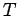is the temperature,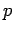andare the pressure and standard pressure,andare the mole number and mole fraction, respectively, of chemical speciesin phase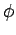,andare the chemical potential and chemical potential at the standard pressure, respectively, andis the gas constant [see also Sugiyama et al., 2001]. Here, the gas phase is indicated by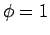.is the Kronecker delta. We assume that only the chemical potential of the gas phase depends on pressure.

The chemical potential of each gas species at the standard pressure is calculated from the following equation: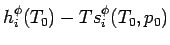whereis the standard temperature,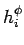is the molecular enthalpy,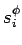is the molecular entropy, and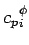is the specific heat at constant pressure. The values of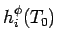,, andare adopted from NIST-JANAF Thermochemical Tables . Spline interpolation is used to give a functional form of. The chemical potential of each condensed species at the standard pressure, except for NH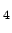SH, is given bywhereis the saturated vapor pressure of species, which is evaluated by the Antoine equation [Chemical Handbook, 1993]. The values of Antoine coefficients are adopted from the Chemical Handbook . In the lower temperature range, where the Antoine equation is not valid,is given as a quadratic function of temperature that is smoothly connected to the above expression. The chemical potential of the condensed phase of NHSH at the standard pressure is calculated from the equilibrium constant for NHSH formation reaction [Lewis, 1969].

The RAND method [White et al., 1953; Van Zeggeren and Storey, 1970, Wood and Hashimoto, 1993] is used to obtain equilibrium composition. During the application of the RAND method, we examine whether or not each condensed phase really equilibrates with the atmospheric gas phase under the given temperature and pressure. This check of phase stability is required in order to ensure non-singularity to the coefficient matrix of the RAND method, to avoid an optimized solution converging to local minimum, and to accelerate the execution speed of numerical calculation of the RAND method. Our source code is available at http://www.gfd-dennou.org/library/oboro/.

## 1.2 Moist adiabatic profile

The vertical profiles of temperature, composition, and condensates are obtained by considering an air parcel following the pseudo moist adiabatic process. We assume that all of the condensates are removed from the air parcel, while the value of total entropy, which is the sum of those for gas and already removed condensates at each pressure level, is conserved.

## 1.3 Static stability

The value ofis estimated as described by Achterberg and Ingersoll . The profiles of the temperature and mean molecular weight are those obtained for the pseudo moist adiabatic process. The value ofis then given as follows:(1)

whereis the acceleration of gravity,is the mean molecular weight, and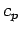is the mean specific heat per mole. The interpretation of the moist adiabat as a rough proxy of the mean atmospheric thermal structure is based on the knowledge of the earth's troposphere (e.g., Gill ). Although dynamics may alter the detailed profiles ofand, as is exemplified by Nakajima et al. , we believe that this formula is still applicable to arguments concerning the order of magnitude.

Thegiven by equation () is slightly different from that of Achterberg and Ingersoll  with respect to the definition of specific heat. We herein adopt the mean specific heat of an air parcel at a given pressure level, whereas Achterberg and Ingersoll  adopt that of a condensible-free air parcel. They assume that the abundances of any condensible elements in the Jovian atmospheres are sufficiently small. Our value ofis approximately the same as that of Achterberg and Ingersoll  in the parameter ranges considered in their study.

# 2 References

Achterberg, R. K., and A. P. Ingersoll (1989), A Normal-Mode Approach to Jovian Atmosphere Dynamics, J. Atmos. Sci., 46, 2448-2462.

Atreya, S. K., and P. N. Romani (1985), Photochemistry and clouds of Jupiter, Saturn and Uranus, in Recent Advances in Planetary Meteorology, edited by G. E. Hunt, pp. 17-68, Cambridge Univ. Press, London.

Chase, M. W. (Eds.) (1989), NIST-JANAF Thermochemical Tables, 4th ed., AIP Press, New York.

Gill, A. E., (1982), Atmosphere-Ocean Dynamics, Academic Press, SanDiego.

Ingersoll, A. P., H. Kanamori, and T. E. Dowling (1994), Atmospheric gravity waves from the impact of comet Shoemaker-Levy 9 with Jupiter, Geophys. Res. Lett., 21, 1083-1086.

The Chemical Society of Japan (Eds.) (1993), Chemical Handbook (Kagaku-Binran), 4th ed., Maruzen, Tokyo, in Japanese.

Lewis, J. S. (1969), The Clouds of Jupiter and the NH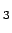-HO and NH-HS Systems, Icarus, 10, 365-378.

Nakajima, K., S. Takehiro, M. Ishiwatari, and Y.-Y. Hayashi (2000), Numerical modeling of Jupiter's moist convection layer, Geophys. Res. Lett., 27, 3129-3133.

Sugiyama, K., M. Odaka, K. Kuramoto, and Y.-Y. Hayashi (2001), Thermodynamic calculation of the atmosphere of the Jovian Planets, Proceedings of the 34 th ISAS Lunar and Planetary symposium, 53-56.

Van Zeggeren, F., and S. H. Storey (1970), The Computation of Chemical Equilibria, Cambridge Univ. Press, London.

Weidenschilling, S. J. and J. S. Lewis (1973), Atmospheric and cloud structure of the Jovian planet, Icarus, 20, 465-476.

White W. B., S. M. Johnson, and G. B. Dantxig (1953), Chemical Equilibrium in Complex Mixture, J. Chem. Phys., 28, 751-755.

Wood, J.A. and A. Hashimoto (1993), Mineral equilibrium in fractionated nebular systems, Geochimica et Cosmochimica Acta, 57, 2377-2388

# $B$3$NJ8=q$K$D$$F(B... B3NJ8=qO(BLaTeX2HTML BK]Lu%W%m%0%i%`(B Version 2002-2-1 (1.70) Copyright © 1993, 1994, 1995, 1996, Nikos Drakos, Computer Based Learning Unit, University of Leeds, Copyright © 1997, 1998, 1999, Ross Moore, Mathematics Department, Macquarie University, Sydney. Br(BBF|K\8l2=7?bN(B( 2002-2-1 (1.70) JA patch-1.8 BHG(B) Copyright © 1998, 1999, Kenshi Muto, Debian Project. Copyright © 2001, 2002, Shige TAKENO, Niigata Inst.Tech. BrMQ$$$F@8@.$5$l$^$7$?!#(B $B%3%^%s%I9T$O0J2<$NDL$j$G$7$?!#(B:
latex2html -no_footnode -local_icons -short_extn -bottom_navigation -no_contents_in_navigation -no_auto_link -up_url /arch/deepconv/index.htm -up_title deepconv -split 3 -show_section_numbers oboro.tex.

$BK]Lu$O(B SUGIYAMA Ko-ichiro $B$K$h$C$F(B $BJ?@.(B18$BG/(B2$B7n(B2$BF|(B $B$K: deepconv SUGIYAMA Ko-ichiro $BJ?@.(B18$BG/(B2$B7n(B2\$BF|(B